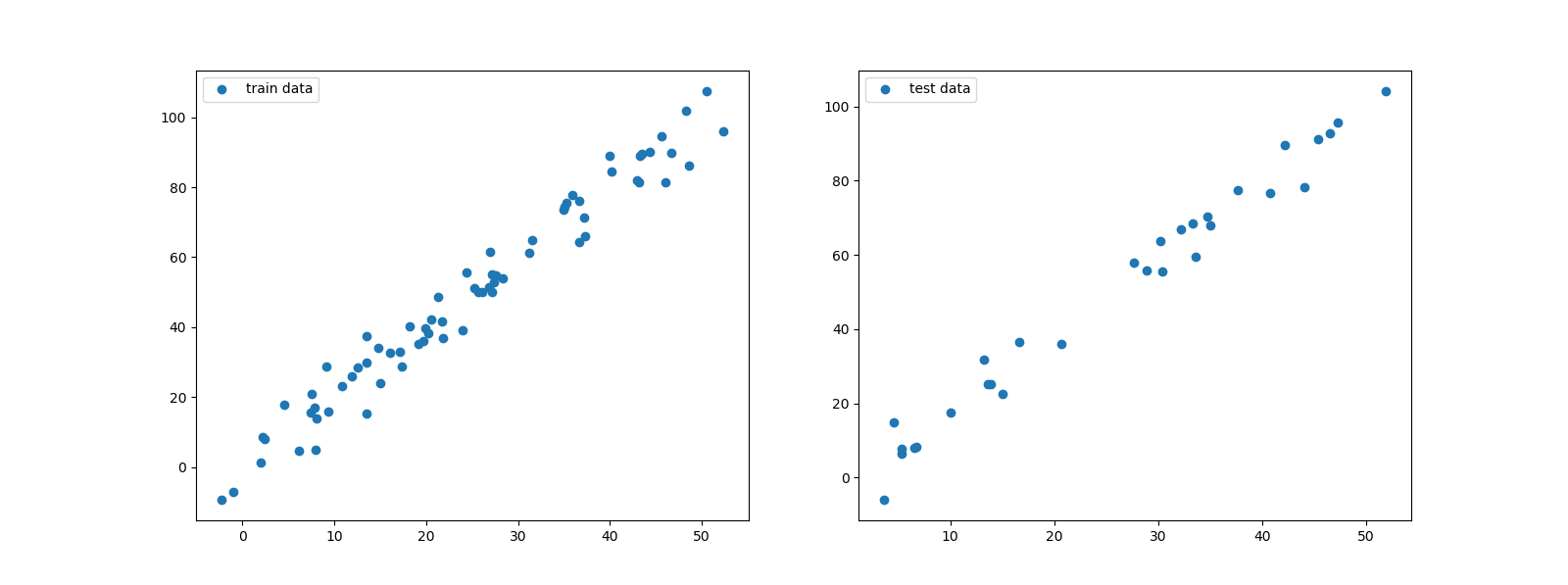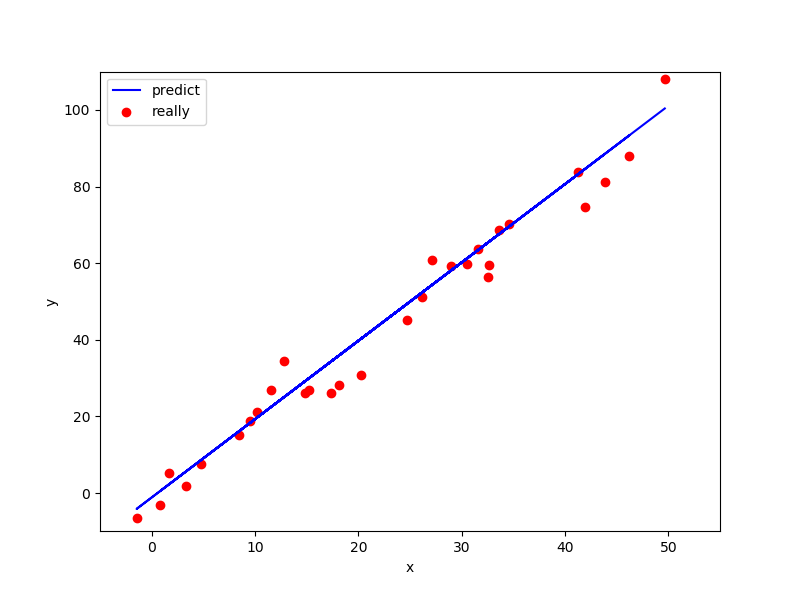## 机器学习-逻辑回归与线性回归

logistic回归与线性回归实际上有很多相同之处，最大的区别就在于他们的因变量不同，其他的基本都差不多，正是因为如此，这两种回归可以归于同一个家族，即广义线性模型（generalized
linear
model）。这一家族中的模型形式基本上都差不多，不同的就是因变量不同，如果是连续的，就是多重线性回归，如果是二项分布，就是logistic回归。logistic回归的因变量可以是二分类的，也可以是多分类的，但是二分类的更为常用，也更加容易解释。所以实际中最为常用的就是二分类的logistic回归。而逻辑回归是用于分类问题，线性回归才是用于回归问题的。

```#第二天 逻辑回归与线性回归
#导入iris数据
from sklearn.datasets import load_iris
#导入回归方法
from sklearn.linear_model import LinearRegression,LogisticRegression
#导入拆分数据集的方法
from sklearn.model_selection import train_test_split
#用于分析验证测试结果
from sklearn.metrics import confusion_matrix,classification_report

# 载入sklearn数据
iris = load_iris()
# 获取特征数据
iris_data = iris['data']
# 特征列名
columns = iris['feature_names']
# 获取签值
iris_label = iris['target']

# 拆分训练集和测试集
train_x,test_x,train_y,test_y = train_test_split(iris_data,iris_label,test_size=0.3)
clf = LogisticRegression()
#训练
clf.fit(train_x,train_y)
#预测
predict_y = clf.predict(test_x)

print(confusion_matrix(test_y,predict_y))
print(classification_report(test_y,predict_y))```

```[[18  0  0]
[ 0 13  1]
[ 0  1 12]]```

```             precision    recall  f1-score   support

0       1.00      1.00      1.00        18
1       0.93      0.93      0.93        14
2       0.92      0.92      0.92        13

avg / total       0.96      0.96      0.96        45```

```import numpy as np
from matplotlib import pyplot as plt

#生成二维的线性散点，加入一些偏移量
x = np.linspace(0, 50, 100) + 2*np.random.randn(100)
y = 2*x + 5*np.random.randn(100)
#预测需要的x值二维数组，用此方法将数组转换成二维的
x = x.reshape(-1,1)
# 上面例子有，同样是拆分训练集和测试集
train_x,test_x,train_y,test_y = train_test_split(x,y,test_size=0.3)
# 我们先画一下训练集和测试集的数据吧
plt.figure(figsize=(16,6))
plt.subplot(1,2,1)
plt.scatter(train_x,train_y,label='train data')
plt.legend()
plt.subplot(1,2,2)
plt.scatter(test_x,test_y,label='test data')
plt.legend()
plt.show()``````# 线性回归方法
clf = LinearRegression()
# 用训练集训练
clf.fit(train_x,train_y)
# 预测测试集
predict_y = clf.predict(test_x)
# 打印出来
plt.figure(figsize=(8,6))
plt.xlim([-5,55])
plt.ylim([-10,110])
plt.xlabel('x')
plt.ylabel('y')
plt.scatter(test_x,test_y,c='r',label='really')
plt.plot(test_x,predict_y,color='b',label='predict')
plt.legend()
plt.show()```0条评论